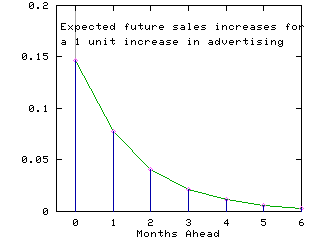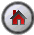SHAZAM Lagged Variables

## Working with lagged variables

Regression equations that use time series data often contain lagged variables. For example, consider the regression equation:

Yt =0 +1 Yt`-`1 +2 Xt + et     for t = 2,...,T

where et is a random error term and the total number of observations in the data set is T. This equation contains a lagged dependent variable as an explanatory variable. This is called an autoregressive model or a dynamic model. Note that the sample period is adjusted to start at observation 2. This is because the first observation is "lost" when a lagged variable is required. So the estimation now uses T`-`1 observations.

Another example of a model with lagged variables is:

Yt =0 +1 Xt +2 Xt`-`1 +3 Xt`-`2 +4 Xt`-`3 + ut     for t = 4,...,T

This model includes current and lagged values of the explanatory variables as regressors. This is called a distributed-lag model.

In SHAZAM lagged variables are created by using the `GENR` command with the `LAG` function. For a 1-period lag, the command format is:

 ` GENR newvar=LAG(var)`

In general, for an n-period lag, the command format is:

 ` GENR newvar=LAG(var,n)`

where `n` is the number of lags required.

Some important rules must be followed when the `LAG` function is used.

1. When lags are taken SHAZAM typically sets the initial undefined observations to 0. Therefore, the `SAMPLE` command must be adjusted to ensure that the subsequent analysis will not include the 0 observations.

2. The time series data must be ordered with the earliest observation as the first observation and the most recent observation as the final observation in the data set.

3. If the left-hand side variable has the same name as the variable in the `LAG` function then a recursive calculation is implemented. For example, suppose capital stock is to be computed as:
```         CAPITAL(t) = CAPITAL(t-1) + INVEST(t)
```

and the initial capital stock is `25.3`. The capital stock series can be computed with the SHAZAM commands:

 ```GENR CAPITAL=25.3 SAMPLE 2 T GENR CAPITAL=LAG(CAPITAL)+INVEST ```

#### Example - Regression with a Lagged Dependent Variable

This example uses a data set on monthly sales and advertising expenditures of a dietary weight control product. It is expected that the impact of advertising expenditures (variable name ADVERT) on sales (variable name SALES) will be distributed over a number of months. A model that captures the lagged advertising effects is:

SALESt =+SALESt`-`1 +ADVERTt + et     for   t = 2,...,T

The coefficients,, andcan be estimated by the method of ordinary least squares. However, the presence of the lagged dependent variable means that the OLS estimation rule does not give a linear unbiased estimator. It follows that hypothesis testing will only be approximately valid. A result that can be established is that if the error process is serially uncorrelated then the lagged dependent variable will be uncorrelated with the current period error and the OLS estimator will be consistent (close to the true parameter value with high probability in large samples).

By repeated substitution for SALESt`-`1 it is found that an increase of 1 unit in advertising in month t leads to an increase in sales of:in period t,in period t+1,2 in period t+2,3 in period t+3,   etc.

With || < 1 this gives a pattern of exponentially declining impacts as time goes on. The total increase in sales over all current and future time periods is the sum:(1 ++2 +3 + . . . )   =`/` (1 `-`)

This is the result for the sum of an infinite geometric series when || < 1. After only k time periods the effect is:(1 ++ . . . +k )   =(1 `-`k+1 ) `/` (1 `-`)

Thus at time k, the percentage of the total advertising effect realized is:

100 (1 `-`k+1 ) %

The above can be solved to find the time period k at which 100p percent of the impact on sales is expected. This gives:

k = log(1 `-` p) `/` log() ` - ` 1

The SHAZAM commands (filename: `SALES.SHA`) for equation estimation and analysis of the results follow.

 ```SAMPLE 1 36 READ (SALES.txt) SALES ADVERT GENR L1SALES=LAG(SALES) * List the data and take a look PRINT SALES L1SALES ADVERT * Adjust the sample period SAMPLE 2 36 OLS SALES L1SALES ADVERT / COEF=BETA * Analyze the effect of a 1 unit increase in advertising. GEN1 A=BETA:1 GEN1 B=BETA:2 * Get the total impact of advertising on all future sales. GEN1 TOTAL = B/(1-A) * Find the time period at which 95% of the impact is expected. GEN1 P95 = LOG(1-.95)/LOG(A) - 1 PRINT TOTAL P95 * Find the expected increases in sales for up to 6 months ahead. SAMPLE 1 7 GENR AHEAD=TIME(-1) GENR IMPACT = B*(A**AHEAD) PRINT AHEAD IMPACT STOP ```

The SHAZAM output can be viewed. The estimated equation is:

SALESt = 7.45 + 0.528 SALESt`-`1 + 0.146 ADVERTt + êt

The results show that a 1 unit increase in advertising gives a 0.146 unit increase in sales in the current month. However, the total expected increase in sales in the current and all future months is calculated as:

```         0.146 / (1 - 0.528) =  0.310
```

The time period at which 95% of the effect is realized is found as:

```         log(1 - 0.95)/log(0.528) - 1  =  3.69
```

This implies that after 4 months more than 95% of the advertising effect will be reflected in the sales performance. The figure below shows the month by month sales response to advertising in the current month.[SHAZAM Guide home]

#### SHAZAM output - Regression with a Lagged Dependent Variable

``` |_SAMPLE 1 36

UNIT 88 IS NOW ASSIGNED TO: SALES.txt
2 VARIABLES AND       36 OBSERVATIONS STARTING AT OBS       1

|_GENR L1SALES=LAG(SALES)
..NOTE.LAG VALUE IN UNDEFINED OBSERVATIONS SET TO ZERO
|_* List the data and take a look
12.00000       .0000000       15.00000
20.50000       12.00000       16.00000
21.00000       20.50000       18.00000
15.50000       21.00000       27.00000
15.30000       15.50000       21.00000
23.50000       15.30000       49.00000
24.50000       23.50000       21.00000
21.30000       24.50000       22.00000
23.50000       21.30000       28.00000
28.00000       23.50000       36.00000
24.00000       28.00000       40.00000
15.50000       24.00000       3.000000
17.30000       15.50000       21.00000
25.30000       17.30000       29.00000
25.00000       25.30000       62.00000
36.50000       25.00000       65.00000
36.50000       36.50000       46.00000
29.60000       36.50000       44.00000
30.50000       29.60000       33.00000
28.00000       30.50000       62.00000
26.00000       28.00000       22.00000
21.50000       26.00000       12.00000
19.70000       21.50000       24.00000
19.00000       19.70000       3.000000
16.00000       19.00000       5.000000
20.70000       16.00000       14.00000
26.50000       20.70000       36.00000
30.60000       26.50000       40.00000
32.30000       30.60000       49.00000
29.50000       32.30000       7.000000
28.30000       29.50000       52.00000
31.30000       28.30000       65.00000
32.30000       31.30000       17.00000
26.40000       32.30000       5.000000
23.40000       26.40000       17.00000
16.40000       23.40000       1.000000

|_SAMPLE 2 36
|_OLS SALES L1SALES ADVERT / COEF=BETA

OLS ESTIMATION
35 OBSERVATIONS     DEPENDENT VARIABLE = SALES
...NOTE..SAMPLE RANGE SET TO:    2,   36

R-SQUARE =    .6720     R-SQUARE ADJUSTED =    .6515
VARIANCE OF THE ESTIMATE-SIGMA**2 =   12.142
STANDARD ERROR OF THE ESTIMATE-SIGMA =   3.4845
SUM OF SQUARED ERRORS-SSE=   388.53
MEAN OF DEPENDENT VARIABLE =   24.606
LOG OF THE LIKELIHOOD FUNCTION = -91.7859

VARIABLE   ESTIMATED  STANDARD   T-RATIO        PARTIAL STANDARDIZED ELASTICITY
NAME    COEFFICIENT   ERROR      32 DF   P-VALUE CORR. COEFFICIENT  AT MEANS
L1SALES    .52793      .1021       5.170      .000  .675      .5478      .5252
ADVERT     .14647      .3308E-01   4.428      .000  .616      .4692      .1721
CONSTANT   7.4469      2.470       3.015      .005  .470      .0000      .3027

|_* Analyze the effect of a 1 unit increase in advertising.
|_GEN1 A=BETA:1
|_GEN1 B=BETA:2

|_*   Get the total impact of advertising on all future sales.
|_GEN1 TOTAL = B/(1-A)
|_*   Find the time period at which 95% of the impact is expected.
|_GEN1 P95 = LOG(1-.95)/LOG(A) - 1
|_PRINT TOTAL P95
TOTAL
.3102750
P95
3.689629

|_*   Find the expected increases in sales for up to 6 months ahead.
|_SAMPLE 1 7[SHAZAM Guide home]